### Geometry Class 9th Mathematics Term 3 Tamilnadu Board Solution

##### Question 1.O is the centre of the circle. AB is the chord and D is mid-point of AB. If the length of CD is 2cm and the length of chord is12 cm, what is the radius of the circle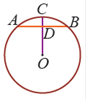A. 10cmB. 12cmC. 15cmD. 18cmAnswer:In triangle AODAD = 6cmAO = rOD = OC–CD = r–2Applying Pythagoras theorem we get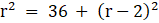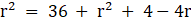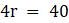r = 10cmQuestion 2.ABCD is a cyclic quadrilateral. Given that ∠ADB + ∠DAB = 120°and ∠ABC + ∠BDA = 145°. Find the value of ∠CDB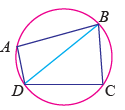A. 75°B. 115°C. 35°D. 45°Answer:As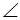ADB +DAB = 120°In triangle ABD as sum of all angles = 180°ABD = 180–120 = 60°As ABCD is cyclic, opposite angles have sum of 180°LetBAD = x°HenceBCD = 180–xLetBDC = a°LetDBC = z°;ABC = 60 + zLetADB = y°As sum of angles of triangle BDC = 180°180–x + a + z = 180a = x–z ...(1)According to question,x + y = 120 ..(2)and60 + z + y = 145...(3)Subtracting (3) from (2)We getx + y–60–z–y = –25x–z = 35Equating from (1) we geta = 35°henceCDB = 35°Question 3.In the given figure, AB is one of the diameters of the circle and OC is perpendicular to it through the center O. If AC is 7√2 cm, then what is the area of the circle in cm2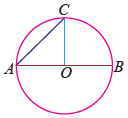A. 24.5B. 49C. 98D. 154Answer:In triangle AOC AO = OC = radius of circle = rUsing Pythagoras theorem,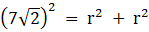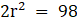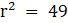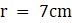As area of a circle is given by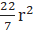=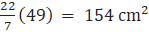Question 4.In the given figure, AB is a diameter of the circle and points C and D are on the circumference such that ∠CAD = 30°and ∠CBA = 70° what is the measure of ACD?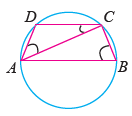A. 40°B. 50°C. 30°D. 90°Answer:According to theorem the angle subtended by a diameter on the circumference of a circle is 90°ACB = 90°Angle subtended by chord AC on major segment =ABC = 70°Angle subtended on minor segment = 180–70 = 110° =ADCAs sum of angles of triangle ADC = 180°ACD = 180–30–110 = 40°Question 5.Angle in a semi circle isA. obtuse angleB. right angleC. an acute angleD. supplementaryAnswer:As a semicircle is formed by a diameter and the angle subtended on the circumference by the diameter is 90°.Question 6.Angle in a minor segment isA. an acute angleB. an obtuse angleC. a right angleD. a reflexive angleAnswer:The angle formed by a chord in the major segment is acute whereas in the minor segment is obtuse and the sum of both angles is 180°.Question 7.In a cyclic quadrilateral ABCD, ∠A = 5x, ∠C = 4x the value of x isA. 12°B. 20°C. 48°D. 36°Answer:In a cyclic quadrilateral sum of opposite angles is 180°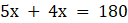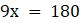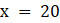Question 8.Angle in a major segment isA. an acute angleB. an obtuse angleC. a right triangleD. a reflexive angleAnswer:The angle formed by a chord in the major segment is acute whereas in the minor segment is obtuse and the sum of both angles is 180°.Question 9.If one angle of a cyclic quadrilateral is 70°, then the angle opposite to it isA. 20°B. 110°C. 140°D. 160°Answer:As the sum of opposite angles in a cyclic quadrilateral is 180°Let opposite angle be x°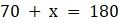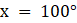PDF FILE TO YOUR EMAIL IMMEDIATELY PURCHASE NOTES & PAPER SOLUTION. @ Rs. 50/- each (GST extra)

HINDI ENTIRE PAPER SOLUTION

MARATHI PAPER SOLUTION

SSC MATHS I PAPER SOLUTION

SSC MATHS II PAPER SOLUTION

SSC SCIENCE I PAPER SOLUTION

SSC SCIENCE II PAPER SOLUTION

SSC ENGLISH PAPER SOLUTION

SSC & HSC ENGLISH WRITING SKILL

HSC ACCOUNTS NOTES

HSC OCM NOTES

HSC ECONOMICS NOTES

HSC SECRETARIAL PRACTICE NOTES

# 2019 Board Paper Solution

HSC ENGLISH SET A 2019 21st February, 2019

HSC ENGLISH SET B 2019 21st February, 2019

HSC ENGLISH SET C 2019 21st February, 2019

HSC ENGLISH SET D 2019 21st February, 2019

SECRETARIAL PRACTICE (S.P) 2019 25th February, 2019

HSC XII PHYSICS 2019 25th February, 2019

CHEMISTRY XII HSC SOLUTION 27th, February, 2019

OCM PAPER SOLUTION 2019 27th, February, 2019

HSC MATHS PAPER SOLUTION COMMERCE, 2nd March, 2019

HSC MATHS PAPER SOLUTION SCIENCE 2nd, March, 2019

SSC ENGLISH STD 10 5TH MARCH, 2019.

HSC XII ACCOUNTS 2019 6th March, 2019

HSC XII BIOLOGY 2019 6TH March, 2019

HSC XII ECONOMICS 9Th March 2019

SSC Maths I March 2019 Solution 10th Standard11th, March, 2019

SSC MATHS II MARCH 2019 SOLUTION 10TH STD.13th March, 2019

SSC SCIENCE I MARCH 2019 SOLUTION 10TH STD. 15th March, 2019.

SSC SCIENCE II MARCH 2019 SOLUTION 10TH STD. 18th March, 2019.

SSC SOCIAL SCIENCE I MARCH 2019 SOLUTION20th March, 2019

SSC SOCIAL SCIENCE II MARCH 2019 SOLUTION, 22nd March, 2019

XII CBSE - BOARD - MARCH - 2019 ENGLISH - QP + SOLUTIONS, 2nd March, 2019

# HSCMaharashtraBoardPapers2020

(Std 12th English Medium)

HSC ECONOMICS MARCH 2020

HSC OCM MARCH 2020

HSC ACCOUNTS MARCH 2020

HSC S.P. MARCH 2020

HSC ENGLISH MARCH 2020

HSC HINDI MARCH 2020

HSC MARATHI MARCH 2020

HSC MATHS MARCH 2020

# SSCMaharashtraBoardPapers2020

(Std 10th English Medium)

English MARCH 2020

HindI MARCH 2020

Hindi (Composite) MARCH 2020

Marathi MARCH 2020

Mathematics (Paper 1) MARCH 2020

Mathematics (Paper 2) MARCH 2020

Sanskrit MARCH 2020

Sanskrit (Composite) MARCH 2020

Science (Paper 1) MARCH 2020

Science (Paper 2)

Geography Model Set 1 2020-2021

MUST REMEMBER THINGS on the day of Exam

Are you prepared? for English Grammar in Board Exam.

Paper Presentation In Board Exam

How to Score Good Marks in SSC Board Exams

Tips To Score More Than 90% Marks In 12th Board Exam

How to write English exams?

How to prepare for board exam when less time is left

How to memorise what you learn for board exam

No. 1 Simple Hack, you can try out, in preparing for Board Exam

How to Study for CBSE Class 10 Board Exams Subject Wise Tips?

JEE Main 2020 Registration Process – Exam Pattern & Important Dates

NEET UG 2020 Registration Process Exam Pattern & Important Dates

How can One Prepare for two Competitive Exams at the same time?

8 Proven Tips to Handle Anxiety before Exams!